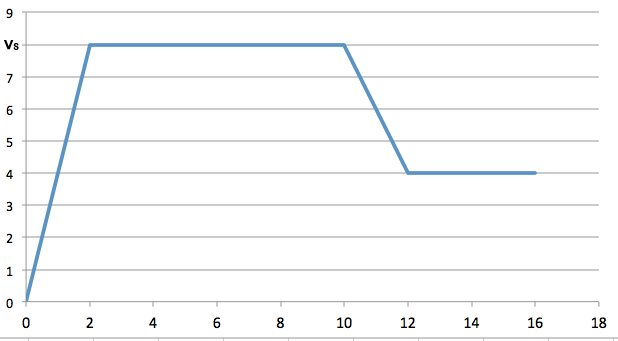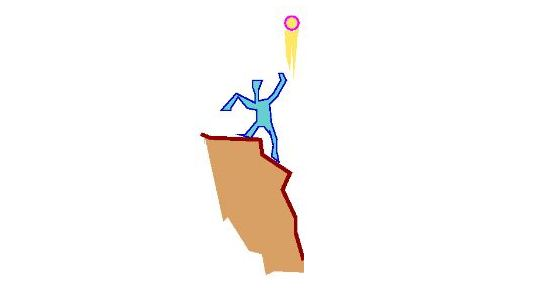Calculus

# Finding Displacement given Velocity

A particle moves along the $x$-axis with velocity $v(t)=30t^2-90t+60$ at time $t$. Find the total distance covered between time $t=0$ and time $t=5.$The above is the velocity-time graph of a runner. How far does this runner travel for $16$ seconds?

The figure’s vertical scaling is set by $v_{s} = 8.0 \text{ m/s.}$

A train starts from station $A$ and arrives at station $B$ in $6$ minutes. If the velocity of the train in $\text{m/min}$ is $v(t) = 24t^2(6-t),$ what is the distance between the two stations $A$ and $B?$Sam throws a ball straight upward at a speed of $30 \text{ m/s}$ from the edge of a cliff $19 \text{ m}$ high, as shown in the above diagram. If the the velocity of the ball $t$ seconds after the ball leaves his hand is $v=30-10t$ (in $\text{m/s}$), what is the distance of the ball from the ground $3$ seconds after the ball leaves his hand?

Two points $P$ and $Q$ start at the origin and move from the origin at the same time along the $x$-axis. At time $t>0$, $P$ and $Q$ have velocities of $\sin (\pi t)$ and $2\sin (2\pi t)$, respectively. If $P$ and $Q$ first meet again after $\frac{m}{n}$ seconds, where $m$ and $n$ are positive co-prime integers, what is the value of $m+n$?

×